# Explain the Armstrong Method for the Generation of FM

### Armstrong Method for the Generation of FM

In the direct methods of generation of FM, LC oscillators are to be used. The crystal oscillator cannot be used. The LC oscillators are not stable enough for the communication or broadcast purpose. Thus, the direct methods cannot be used for the broadcast applications.

The alternative method is to use the indirect method called as the Armstrong method of FM generation.

In this method, the FM is obtained through phase modulation. A crystal oscillator can be used hence the frequency stability is very high and this method is widely used in practice.

Figure.1 shows the block diagram of the Armstrong method.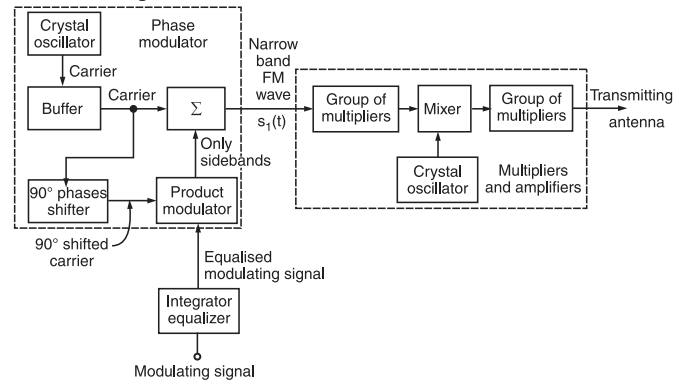Fig.1 : Armstrong Method for FM Generation

The Armstrong method uses the phase modulator to generate a frequency modulated wave.

### Working Principle

The working operation of this system can be divided into two parts as follows:

Part I: Generate a narrow band FM wave using a phase modulator.

Part II: Use the frequency multipliers and mixer to obtain the required values of frequency deviation, carrier and modulation index.

### Part I: Generate a narrow band FM using Phase Modulator

As discussed carrier, we can generate FM using a phase modulator.

The modulating signal x(t) is passed through an integrator before applying it to the phase modulator as shown in figure 1.

Let the narrow band FM wave produced at the output of the phase modulator be represented by s1(t) i.e.,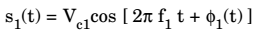where Vc1 is the amplitude and f1 is the frequency of the carrier produced by the crystal oscillator.

The phase angle Φ1(t) of s1(t) is related to x(t) as follows: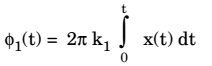where k1 represents the frequency sensitivity of the modulator.

If Φ1(t) is very small then,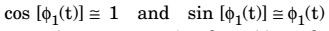Hence, the approximate expression for s1(t) can be obtained as follows: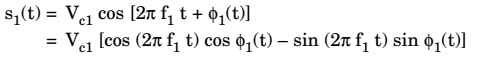After approximation, we get,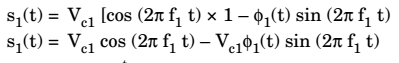Substituting,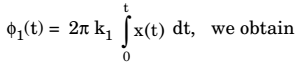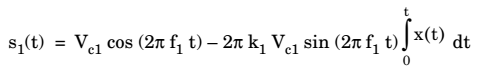This expression represents a narrow band FM. Thus, at the output of the phase modulator, we obtain a narrow band FM wave.

### Part II: Implementation of the Phase Modulator

Figure.2  shows the block diagram of phase modulator circuit.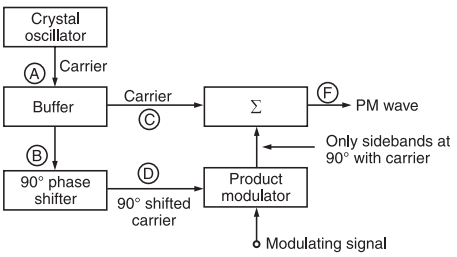Fig.2 : Phase Modulator Circuit

### Working Principle

The crystal oscillator produces a stable unmodulated carrier which is applied to the 90° phase shifter as well as the combining network through a buffer.

The 90° phase shifter produces a 90° phase shifted carrier. It is applied to the balanced modulator along with the modulating signal.

Thus, the carrier used for modulation is 90° shifted with respect to the original carrier.

At the output of the product modulator, we get DSB SC signal i.e., AM signal without carrier.

This signal consists of only two sidebands with their resultant in phase with the 90° shifted carrier .

The two sidebands and the original carrier without any phase shift are applied to a combining network (∑). At the output of the combining network, we get the resultant of vector addition of the carrier and two sidebands as shown in figure 2.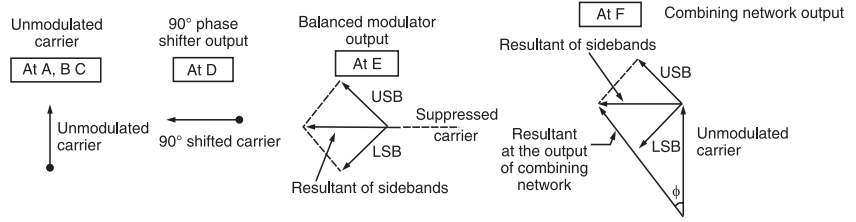Fig.2: Phasors explaining the generation of PM

Now, as the modulation index is increased, the amplitude of sidebands will also increase. Hence, the amplitude of their resultant increases. This will increase the angle Φ made by the resultant with unmodulated carrier.

The angle Φ decreases with reduction in modulation index as shown in figure 3.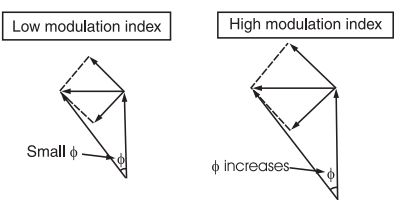Fig.3 : Effect of modulation index on frequency f

Thus, the resultant at the output of the combining network is phase modulated. Hence, the block diagram of figure.1 operates as a phase modulator.

### Part III: Use of Frequency Multipliers Mixer and Amplifier

The FM signal produced at the output of phase modulator has a low carrier frequency and low modulation index. They are increased to an adequately high value with the help of frequency multipliers and mixer.